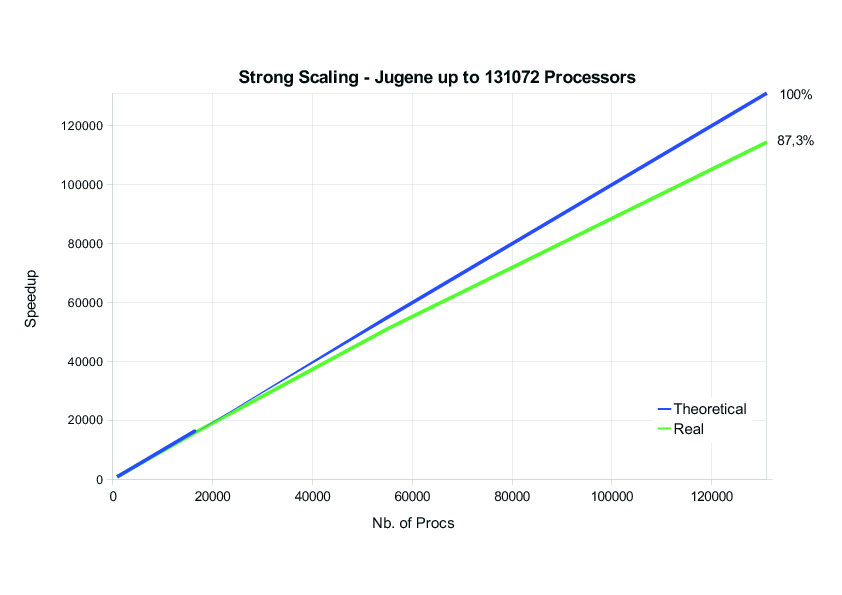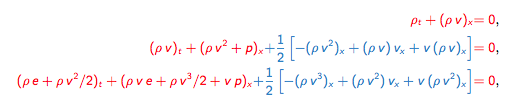Research

For more actual information about the research activities, please visit the publication section.

The research group ‚Numerical Simulation’ of the Mathematical Insitute at University of Colgone works on the development and analysis of efficient numerical methods for the simulation of problems in natural sciences and engineering.

The focus is on the development of high order methods for hyperbolic and mixed hyperbolic/parabolic partial differential equations with a special emphasis on high performance computing. Applications include e.g. the compressible Navier-Stokes equations (turbulence), the ideal and resistive Magnetohydrodynamic equations (plasma) and the Shallow Water equations (ocean surface waves).

The goal is to develop novel numerical schemes and algorithms that are effective on over 100,000 processors and beyond. For this, numerical simulation relates several fields such as numerical analysis, performance engineering, data science and physics/engineering.

This plot shows the strong scaling of the method on the former Blue-Gene cluster „Jugene“ of the Jülich Supercomputing Center on up to 131k cores. The computation simulated the fully compressible three-dimensional Navier-Stokes equations using a high order (N=7) discontinuous Galerkin scheme. The excellent scaling of the discontinuous Galerkin scheme is demonstrated, as the total amount of elements for this computation was 131k. Thus, for the highest number of processors, only one element on each processor was left. Despite this, the code stilled reached a scaling of 87%.A recent topic is the design and analysis of skew-symmetric and/or entropy stable discontinuous Galerkin methods for hyperbolic conservation laws. For this, we rely on a strong connection of the so-called discontinuous Galerkin spectral element scheme with summation-by-parts finite difference schemes. This connection allows us to exploit several strategies originally developed in the finite difference community to construct non-linearly stable discontinuous Galerkin discretisations.

For example, considering the compressible Euler equation in one spatial dimension. The red terms shown below would be the standard form of the Euler equations. However, when adding the blue terms (which are actually zero in the continuous case) and using the resulting system as a base for the discretisation, it is possible to recover kinetic energy stability.Similar developments have led to

• Entropy stable and kinetic energy stable discontinuous Galerkin methods for compressible Euler equations
• Entropy stable and well balanced discontinuous Galerkin methods for Shallow Water equations with discontinuous bottom topography
• Entropy stable and well balanced Finite Volume and discontinuous Galerkin methods for the Shallow Water Magnetohydrodynamic equations
• Entropy stable Finite Volume and discontinuous Galerkin discretisations for the Magnetohydrodynamic equations

Another recent topic is to compare and asses the efficiency of such discretisations for instance for compressible turbulence and extend their applicability to problems in e.g. astrophysics.